Courses

# Test: Communication Systems - 1

## 25 Questions MCQ Test Communication Theory | Test: Communication Systems - 1

Description
This mock test of Test: Communication Systems - 1 for Electronics and Communication Engineering (ECE) helps you for every Electronics and Communication Engineering (ECE) entrance exam. This contains 25 Multiple Choice Questions for Electronics and Communication Engineering (ECE) Test: Communication Systems - 1 (mcq) to study with solutions a complete question bank. The solved questions answers in this Test: Communication Systems - 1 quiz give you a good mix of easy questions and tough questions. Electronics and Communication Engineering (ECE) students definitely take this Test: Communication Systems - 1 exercise for a better result in the exam. You can find other Test: Communication Systems - 1 extra questions, long questions & short questions for Electronics and Communication Engineering (ECE) on EduRev as well by searching above.
QUESTION: 1

### A cordless telephone using separate frequencies for transmission in base and portable units is known as

Solution:

Separate frequencies for transmission from base and portable units allows two way transmission and is called duplex arrangement.

QUESTION: 2

### For attenuation of high frequencies we should use

Solution:

Since Xc for high frequencies is low, the high frequencies are shunted to ground and are not transmitted.

QUESTION: 3

### A modem is classified as low speed if data rate handled is

Solution:

When data rate in bits per second is upto 600, modem is low speed.

QUESTION: 4

VSB modulation is preferred in TV because

Solution:

VSB (vestigial side band) transmission transmits one side band fully and the other side band partially thus, reducing the bandwidth requirement.

QUESTION: 5

A woofer should be fed from the input through a

Solution:

Woofer is a low frequency loud speaker covering the range 16 Hz to 500 Hz.

QUESTION: 6

The colour subcarrier and sidebands produced by its modulation with the chrominance signals are accommodated in the standard channel width by the process of

Solution:

In frequency interleaving the space between bundles is used for colour signal.

QUESTION: 7

In FM signal with a modulation index mf is passed through a frequency tripler. The wave in the output of the tripler will have a modulation index of

Solution:

Frequency multiplier increase the deviation,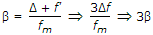.

QUESTION: 8

In colour TV receiver, varactor diode is used for

Solution:

In varactor diode the applied reverse bias controls the width and therefore capacitance of depletion layer.

This capacitance is used for tuning.

QUESTION: 9

The number of noise sources in a BJT are

Solution:

Shot noise, partition noise and thermal noise.

QUESTION: 10

Energy content of atmospheric noise

Solution:

Atmospheric noise decreases as frequency is increased.

QUESTION: 11

A 400 W carrier is amplitude modulated with m = 0.75. The total power in AM is

Solution: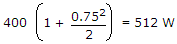.

QUESTION: 12

c(t) and m(t) are used to generate an FM signal. If the peak frequency deviation of the generated FM signal is three times the transmission bandwidth of the AM signal, then the coefficient of the term cos [2p(1008 x 103t)] in the FM signal (in terms of the Bessel Coefficients) is

Solution:

As, we known FM, s(t)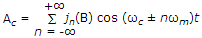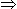Frequency deviation = 3 . (AM Bandwidth) = 6 fm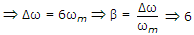and β= 6 is given in (d) only.

ωc + n ωm = (1008 x 103)2p2p x 106 + n. 4p x 103 = (1008 x 103).2pn = 4

So, β = 6, n = 4 hence answer is 5j4(6).

QUESTION: 13

Non-coherently detection is not possible for

Solution:
QUESTION: 14

Tracking of extra terrestrial objects requires

Solution:

All the three are required.

QUESTION: 15

Assertion (A): Free space does not interfere with normal radiation and propagation of radio waves

Reason (R): Free space has no magnetic or gravitational fields.

Solution:

Since free space does not have magnetic and other fields, waves can propagate without any interference.

QUESTION: 16

In radar systems PRF stands for

Solution:

PRF in radar systems means pulse repetition frequency.

QUESTION: 17

Which of the following cannot be the Fourier series expansion of a periodic signal?

Solution:

x(t) = cos t + 0.5

not satisfies the Dirichlet condition.

The integration of constant term is ∞.

QUESTION: 18

Which of the following is the indirect way of FM generation?

Solution:

It generates FM through phase modulation.

QUESTION: 19

A telephone exchange has 9000 subscribers. If the number of calls originating at peak time is 10, 000 in one hour, the calling rate is

Solution:

Calling rate is the number of calls per subscriber.

QUESTION: 20

If C is capacity of noisy channel, (bits/s), δf is bandwidth Hz and S/N is signal to noise ratio, then

Solution:

This is Shannon-Hartley theorem.

QUESTION: 21

As the frequency increases, the absorption of ground wave by earth's surface

Solution:

Because of this reason ground waves can be used for frequencies upto about 1600 kHz only.

QUESTION: 22

The velocity of sound waves in air

Solution:

vT.

QUESTION: 23

The range of a cordless telephone is about

Solution:

The range depends on quality of instrument and is about 100 m.

QUESTION: 24

An earth mat for a communication tower consists of

Solution:

The number of radials is large and depth is about 30 cm.

QUESTION: 25

Degaussing in a picture tube means

Solution:

Since gauss is a unit of magnetic flux, degaussing means removal of magnetism.